# AP Board 8th Class Maths Solutions Chapter 3 Construction of Quadrilaterals Ex 3.2

AP State Syllabus AP Board 8th Class Maths Solutions Chapter 3 Construction of Quadrilaterals Ex 3.2 Textbook Questions and Answers.

## AP State Syllabus 8th Class Maths Solutions 3rd Lesson Construction of Quadrilaterals Exercise 3.2Construct quadrilateral with the measurements given below:

Question (a).
Quadrilateral ABCD with AB = 4.5 cm, BC = 5.5 cm, CD = 4 cm, AD = 6 cm andAC= 7cm
Solution:
In Quadrilateral ABCD with AB = 4.5 cm, BC = 5.5 cm, CD = 4 cm, AD = 6 cm and AC = 7 cm.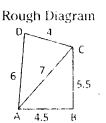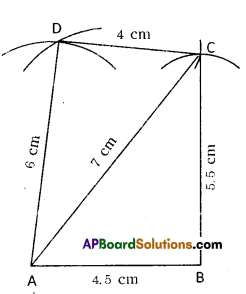Construction Steps:

1. Draw a line segment $$\overline{\mathrm{AB}}$$ with radius 4.5 cms.
2. With the centres A, B draw arcs equal to 7 cm and 5.5 cm respectively. The intersection of these two arcs keep as ‘C’.
3. Join A, C and B, C.
4. With centres C, A draw arcs equal to 4 cm, 6 cm respectively. These intersecting point is keep as ’D’.
Join D, C and A, D.
5. ∴ The required quadrilateral ABCD is formed.Question (b).
Quadrilateral PQRS with PQ = 3.5 cm, QR = 4 cm, RS = 5 cm, PS = 4.5 cm and QS= 6.5 cm
Solution:
PQ = 3.5 cm, QR = 4 cm, RS = 5 cm,
PS – 4.5 cm, QS = 6.5 cm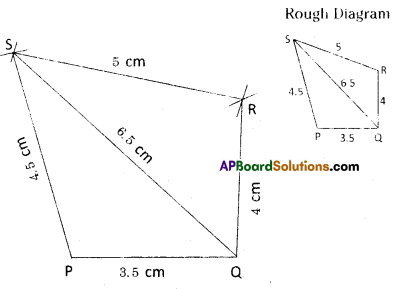Construction Steps:

1. Draw a line segment $$\overline{\mathrm{PQ}}$$ with radius 3.5 cm.
2. With the centres P, Q draw arcs equal to 4.5 cm and 6.5 cm respectively.
3. These two arcs meet at point ‘S’.
4. With the centres S, Q draw arcs with radius 5 cm, 4 cm respectively. These two arcs intersected at point ‘R’.
5. Join P, S; Q, S; S, R and Q, R.
6. ∴ The required quadrilateral PQRS is formed.

Question (c).
Parallelogram ABCD with AB = 6cm, CD = 4.5 cm and BD = 7.5 cm
Solution:
In a parallelogram ABCD; AB = 6 cm, BC = 4.5 cm, BD = 7.5 cm
AB = CD (;cm
BC = AD = 4.5 cm
BD = 7.5 cm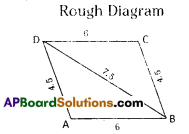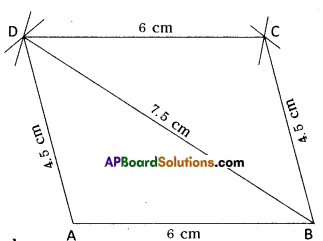Construction Steps:

1. Draw a line segment $$\overline{\mathrm{AB}}$$ with radius 6 cms.
2. With the centres A, B draw arcs with radius 4.5 cm, 7.5 cm respectively. These two arcs meet at point ‘D’.
3. With the centres D, B draw arcs with radius 6 cm, 4.5 cm respectively. These two arcs meet at point ‘C’.
4. Join A, D and B, C and D, C and B, D.
5. ∴ The required parallelogram ABCD is formed.Question (d).
Rhombus NICE with NI = 4 cm and IE = 5.6 cm
Solution:
In a rhombus NI = IC = CE = NE = 4 cm, IE = 5.6 cm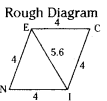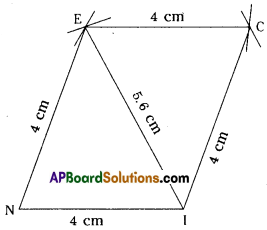Construction Steps:

1. Draw a line segment $$\overline{\mathrm{NI}}$$ with radius 4 cm.
2. With the centres N, I draw two arcs with radius 4 cm, 5.6 cm respectively. These two arcs meet at point E’.
3. With the centres E, I draw arcs with radius 4 cm. These two arcs meet at point ‘C’.
4. Join N, E and I, E. Also join E, C and I, C.
5. .’. The required rhombus NICE is formed.